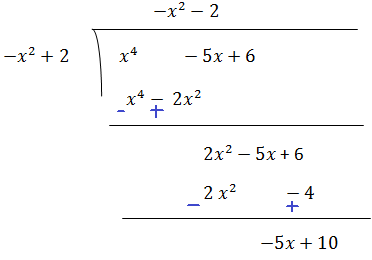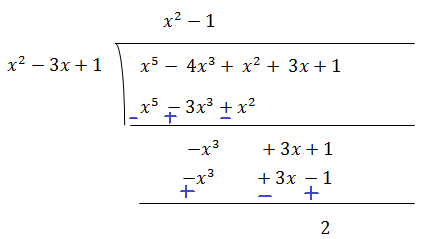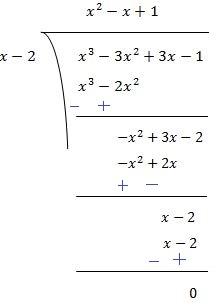# Class 10 Maths Chapter 2 Polynomials

NCERT Solutions for Class 10 Maths Chapter 2 Polynomials. Question Answer.
Page No: 28

Exercises 2.1

1. The graphs of y = p(x) are given in following figure, for some polynomials p(x). Find the number of zeroes of p(x), in each case.(i) The number of zeroes is 0 as the graph does not cut the x-axis at any point.
(ii) The number of zeroes is 1 as the graph intersects the x-axis at only 1 point.
(iii) The number of zeroes is 3 as the graph intersects the x-axis at 3 points.
(iv) The number of zeroes is 2 as the graph intersects the x-axis at 2 points.
(v) The number of zeroes is 4 as the graph intersects the x-axis at 4 points.
(vi) The number of zeroes is 3 as the graph intersects the x-axis at 3 points.

Page No: 33

Exercise 2.2

1. Find the zeroes of the following quadratic polynomials and verify the relationship between the zeroes and the coefficients.
(i) x2 – 2x – 8
(ii) 4s2 – 4s + 1
(iii) 6x2 – 3 – 7x
(iv) 4u2 + 8u
(v) t2 – 15
(vi) 3x2 – x – 4

(i) x2 – 2x – 8
= (x - 4) (x + 2)
The value of x2 – 2x – 8 is zero when x - 4 = 0 or x + 2 = 0, i.e., when x = 4 or x = -2
Therefore, the zeroes of x2 – 2x – 8 are 4 and -2.

Sum of zeroes = 4 + (-2) = 2 = -(-2)/1 = -(Coefficient of x)/Coefficient of x2
Product of zeroes = 4 × (-2) = -8 = -8/1 = Constant term/Coefficient of x2

(ii) 4s2 – 4s + 1
= (2s-1)2
The value of 4s2 - 4s + 1 is zero when 2s - 1 = 0, i.e., s = 1/2
Therefore, the zeroes of 4s2 - 4s + 1 are 1/2 and 1/2.

Sum of zeroes = 1/2 + 1/2 = 1 = -(-4)/4 = -(Coefficient of s)/Coefficient of s2
Product of zeroes = 1/2 × 1/2 = 1/4 = Constant term/Coefficient of s2.

(iii) 6x2 – 3 – 7x
6x– 7– 3
= (3x + 1) (2x - 3)
The value of 6x2 - 3 - 7x is zero when 3x + 1 = 0 or 2x - 3 = 0, i.e., x = -1/3 or x = 3/2
Therefore, the zeroes of 6x2 - 3 - 7x are -1/3 and 3/2.

Sum of zeroes = -1/3 + 3/2 = 7/6 = -(-7)/6 = -(Coefficient of x)/Coefficient of x2
Product of zeroes = -1/3 × 3/2 = -1/2 = -3/6 = Constant term/Coefficient of x2.

(iv) 4u2 + 8u
4u2 + 8u + 0
= 4u(u + 2)
The value of 4u2 + 8u is zero when 4u = 0 or u + 2 = 0, i.e., u = 0 or u = - 2
Therefore, the zeroes of 4u2 + 8u are 0 and - 2.
Sum of zeroes = 0 + (-2) = -2 = -(8)/4 = -(Coefficient of u)/Coefficient of u2
Product of zeroes = 0 × (-2) = 0 = 0/4 = Constant term/Coefficient of u2.

(v) t2 – 15
t- 0.t - 15
= (- √15) (t + √15)
The value of t2 - 15 is zero when t - √15 = 0 or t + √15 = 0, i.e., when t = √15 or = -√15
Therefore, the zeroes of t2 - 15 are √15 and -√15.Sum of zeroes = √15 + -√15 = 0 = -0/1 = -(Coefficient of t)/Coefficient of t2
Product of zeroes = (√15) (-√15) = -15 = -15/1 = Constant term/Coefficient of u2.

(vi) 3x2 – x – 4
= (3x - 4) (x + 1)
The value of 3x2 – x – 4 is zero when 3x - 4 = 0 and x + 1 = 0,i.e., when x = 4/3 or x = -1
Therefore, the zeroes of 3x2 – x – 4 are 4/3 and -1.

Sum of zeroes = 4/3 + (-1) = 1/3 = -(-1)/3 = -(Coefficient of x)/Coefficient of x2
Product of zeroes = 4/3 × (-1) = -4/3 = Constant term/Coefficient of x2.

2. Find a quadratic polynomial each with the given numbers as the sum and product of its zeroes respectively.
(i) 1/4 , -1
(ii) √2 , 1/
(iii) 0, √5
(iv) 1,1
(v) -1/4 ,1/
(vi) 4,1

(i) 1/4 , -1
Let the polynomial be ax2 + bx + c, and its zeroes be α and ß
α + ß = 1/4 = -b/a
αß = -1 = -4/4 = c/a
If a = 4, then b = -1, c = -4
Therefore, the quadratic polynomial is 4x2 - x -4.

(ii) √2 , 1/3
Let the polynomial be ax2 + bx + c, and its zeroes be α and ß
α + ß = √2 = 3√2/3 = -b/a
αß = 1/3 = c/a
If a = 3, then b = -3√2c = 1
Therefore, the quadratic polynomial is 3x2 -3√2x +1.

(iii) 0, √5
Let the polynomial be ax2 + bx + c, and its zeroes be α and ß
α + ß = 0 = 0/1 = -b/a
αß = √5 = √5/1 = c/a
If a = 1, then b = 0, c = √5
Therefore, the quadratic polynomial is x2 + √5.

(iv) 1, 1
Let the polynomial be ax2 + bx + c, and its zeroes be α and ß
α + ß = 1 = 1/1 = -b/a
αß = 1 = 1/1 = c/a
If a = 1, then b = -1, c = 1
Therefore, the quadratic polynomial is x2 - x +1.

(v) -1/4 ,1/4
Let the polynomial be ax2 + bx + c, and its zeroes be α and ß
α + ß = -1/4 = -b/a
αß = 1/4 = c/a
If a = 4, then b = 1, c = 1
Therefore, the quadratic polynomial is 4x2 + x +1.

(vi) 4,1
Let the polynomial be ax2 + bx + c, and its zeroes be α and ß
α + ß = 4 = 4/1 = -b/a
αß = 1 = 1/1 = c/a
If a = 1, then b = -4, c = 1
Therefore, the quadratic polynomial is x2 - 4x +1.

Page No: 36

Exercise 2.3

1. Divide the polynomial p(x) by the polynomial g(x) and find the quotient and remainder in each of the following:

(i) p(x) = x3 – 3x2 + 5x – 3, g(x) = x2 – 2Quotient = x-3 and remainder 7x - 9

(ii) p(x) = x4 – 3x2 + 4x + 5, g(x) = x2 + 1 – xQuotient = x2 + - 3 and remainder 8

(iii) p(x) = x4 – 5x + 6, g(x) = 2 – x2Quotient = -x2 -2 and remainder -5x +10

2. Check whether the first polynomial is a factor of the second polynomial by dividing the
second polynomial by the first polynomial:

(i) t2 – 3,  2t4 + 3t3 – 2t2 – 9t – 12t2 – 3 exactly divides  2t4 + 3t3 – 2t2 – 9t – 12 leaving no remainder. Hence, it is a factor of  2t4 + 3t3 – 2t2 – 9t – 12.

(ii) x2 + 3x + 1, 3x4 + 5x3 – 7x2 + 2x + 2
x2 + 3x + 1 exactly divides 3x4 + 5x3 – 7x2 + 2x + 2 leaving no remainder. Hence, it is factor of 3x4 + 5x3 – 7x2 + 2x + 2.

(iii) x3 – 3x + 1, x5 – 4x3 + x2 + 3x + 1x3 – 3x + 1 didn't divides exactly x5 – 4x3 + x2 + 3x + 1 and leaves 2 as remainder. Hence, it not a factor of x5 – 4x3 + x2 + 3x + 1.

3. Obtain all other zeroes of 3x4 + 6x3 – 2x2 – 10x – 5, if two of its zeroes are √(5/3)
and - √(5/3).

p(x) = 3x4 + 6x3 – 2x2 – 10x – 5
Since the two zeroes are √(5/3) and - √(5/3).We factorize x2 + 2+ 1
= (+ 1)2
Therefore, its zero is given by x + 1 = 0
x = -1
As it has the term (+ 1)2 , therefore, there will be 2 zeroes at x = - 1.
Hence, the zeroes of the given polynomial are √(5/3) and - √(5/3), - 1 and - 1.

4.  On dividing x3 - 3x2 + x + 2 by a polynomial g(x), the quotient and remainder were x - 2 and
-2x + 4, respectively. Find g(x).

Here in the given question,
Dividend = x3 - 3x2 + x + 2
Quotient = x - 2
Remainder = -2x + 4
Divisor = g(x)
We know that,
Dividend = Quotient × Divisor + Remainder
⇒ x3 - 3x2 + x + 2 = (x - 2) × g(x) + (-2x + 4)⇒ x3 - 3x2 + x + 2 - (-2x + 4) = (x - 2) × g(x)
⇒ x3 - 3x2 + 3x - 2 = (x - 2) × g(x)
⇒ g(x) =  (x3 - 3x2 + 3x - 2)/(x - 2)∴ g(x) = (x2 - x + 1)

5.Give examples of polynomial p(x), g(x), q(x) and r(x), which satisfy the division algorithm and
(i) deg p(x) = deg q(x)
(ii) deg q(x) = deg r(x)
(iii) deg r(x) = 0

(i) Let us assume the division of 6x2 + 2x + 2 by 2
Here, p(x) = 6x2 + 2x + 2
g(x) = 2
q(x) = 3x2 + x + 1
r(x) = 0
Degree of p(x) and q(x) is same i.e. 2.
Checking for division algorithm,
p(x) = g(x) × q(x) + r(x)
Or, 6x2 + 2x + 2 = 2x (3x2 + + 1)
Hence, division algorithm is satisfied.

(ii) Let us assume the division of x3x by x2,
Here, p(x) = x3 + x
g(x) = x2
q(x) = x and r(x) = x
Clearly, the degree of q(x) and r(x) is the same i.e., 1.
Checking for division algorithm,
p(x) = g(x) × q(x) + r(x)
x3 + x = (x2 ) × x + x
x3 + x = x3 + x
Thus, the division algorithm is satisfied.

(iii) Let us assume the division of x3+ 1 by x2.
Here, p(x) = x3 + 1
g(x) = x2
q(x) = x and r(x) = 1
Clearly, the degree of r(x) is 0.
Checking for division algorithm,
p(x) = g(x) × q(x) + r(x)
x3 + 1 = (x2 ) × + 1
x3 + 1 = x3 + 1
Thus, the division algorithm is satisfied.

Page No: 36

Exercise 2.4 (Optional)

1. Verify that the numbers given alongside of the cubic polynomials below are their zeroes. Also verify the relationship between the zeroes and the coefficients in each case:
(i) 2x3 + x2 - 5+ 2; 1/2, 1, -2
(ii) x3 - 4x2 + 5x - 2; 2, 1, 1

(i) p(x) = 2x3 + x2 - 5+ 2
Now for zeroes, putting the given value in x.

p(1/2) = 2(1/2)3 + (1/2)2 - 5(1/2) + 2
= (2×1/8) + 1/4 - 5/2 + 2
= 1/4 + 1/4 - 5/2 + 2
= 1/2 - 5/2 + 2 = 0

p(1) = 2(1)3 + (1)2 - 5(1) + 2
= (2×1) + 1 - 5 + 2
= 2 + 1 - 5 + 2 = 0

p(-2) = 2(-2)3 + (-2)2 - 5(-2) + 2
= (2 × -8) + 4 + 10 + 2
= -16 + 16 = 0

Thus, 1/2, 1 and -2 are the zeroes of the given polynomial.

Comparing the given polynomial with ax3 + bx2 + c+ d, we get a=2, b=1, c=-5, d=2
Also, α=1/2, β=1 and γ=-2
Now,
-b/a = α+β+γ
⇒ 1/2 = 1/2 + 1 - 2
⇒ 1/2 = 1/2

c/a = αβ+βγ+γα
⇒ -5/2 = (1/2 × 1) + (1 × -2) + (-2 × 1/2)
⇒ -5/2 = 1/2 - 2 - 1
⇒ -5/2 = -5/2

-d/a = αβγ
⇒ -2/2 = (1/2 × 1 × -2)
⇒ -1 = 1

Thus, the relationship between zeroes and the coefficients are verified.

(ii)  p(x) = x3 - 4x2 + 5x - 2
Now for zeroes, putting the given value in x.

p(2) = 23 - 4(2)2 + 5(2) - 2
= 8 - 16 + 10 - 2
= 0

p(1) = 13 - 4(1)2 + 5(1) - 2
= 1 - 4 + 5 - 2
= 0

p(1) = 13 - 4(1)2 + 5(1) - 2
= 1 - 4 + 5 - 2
= 0

Thus, 2, 1 and 1 are the zeroes of the given polynomial.

Comparing the given polynomial with ax3 + bx2 + c+ d, we get a=1, b=-4, c=5, d=-2
Also, α=2, β=1 and γ=1
Now,
-b/a = α+β+γ
⇒ 4/1 = 2 + 1 + 1
⇒ 4 = 4

c/a = αβ+βγ+γα
⇒ 5/1 = (2 × 1) + (1 × 1) + (1 × 2)
⇒ 5 = 2 + 1 + 2
⇒ 5 = 5

-d/a = αβγ
⇒ 2/1 = (2 × 1 × 1)
⇒ 2 = 2

Thus, the relationship between zeroes and the coefficients are verified.

2. Find a cubic polynomial with the sum, sum of the product of its zeroes taken two at a time, and the product of its zeroes as 2, –7, –14 respectively.

Let the polynomial be ax3 + bx+ cx + d and the zeroes be α, β and γ
Then, α + β + γ = -(-2)/1 = 2 = -b/a
αβ + βγ + γα = -7 = -7/1 = c/a
αβγ = -14 = -14/1 = -d/a

∴ a = 1, b = -2, c = -7 and d = 14
So, one cubic polynomial which satisfy the given conditions will be x3 - 2x2  - 7x + 14

3. If the zeroes of the polynomial x3 – 3x2 + x + 1 are a–b, a, a+b, find a and b.

Since, (a - b), a, (a + b) are the zeroes of the polynomial x3 – 3x2 + x + 1.
Therefore, sum of the zeroes = (a - b) + a + (a + b) = -(-3)/1 = 3

⇒ 3a = 3 ⇒ a =1

∴ Sum of the products of is zeroes taken two at a time = a(a - b) + a(a + b) + (a + b) (a - b) =1/1 = 1
a2 - ab + a2 + ab + a2 - b= 1
⇒ 3a2 - b2 =1

Putting the value of a,

⇒ 3(1)2 - b2 = 1
⇒ 3 - b2 = 1
⇒ b2 = 2
⇒ b = ±√2
Hence, a = 1 and b = ±√2

4. If two zeroes of the polynomial x4 – 6x3 – 26x2 + 138x – 35 are  2±√3,  find other zeroes.

2+√3 and 2-√3 are two zeroes of the polynomial p(x) = x4 – 6x3 – 26x2 + 138x – 35.
Let x = 2±√3
So, x-2 = ±√3
On squaring, we get x2 - 4x + 4 = 3,
⇒ x2 - 4x + 1= 0

Now, dividing p(x) by x2 - 4x + 1∴ p(x) = x4 - 6x3 - 26x2 + 138x - 35
= (x2 - 4x + 1) (x2 - 2x - 35)
= (x2 - 4x + 1) (x2 - 7x + 5x - 35)
= (x2 - 4x + 1) [x(x - 7) + 5 (x - 7)]
= (x2 - 4x + 1) (x + 5) (x - 7)

∴ (x + 5) and (x - 7) are other factors of p(x).
∴ - 5 and 7 are other zeroes of the given polynomial.

5. If the polynomial x4 – 6x3 + 16x2 – 25x + 10 is divided by another polynomial x2 – 2x + k, the remainder comes out to be x + a, find k and a.

On dividing x4 – 6x3 + 16x2 – 25x + 10 by x2 – 2x + k∴ Remainder = (2k - 9)x - (8 - k)k + 10
But the remainder is given as x+ a.

On comparing their coefficients,
2k - 9 = 1
⇒ k = 10
⇒ k = 5 and,

-(8-k)k + 10 = a
⇒ a = -(8 - 5)5 + 10 =- 15 + 10 = -5

Hence, k = 5 and a = -5